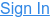Home Blog Release: Crate 0.41.0 (Testing)

# Release: Crate 0.41.0 (Testing)

We're having the honor to introduce you to a new Crate testing release! Version 0.41.0 comes with a brand new set of arithmetic functions for you to crunch and squeeze all the numbers from your tables.

## Introducing Arithmetic Functions

A reference about all implemented arithmetic functions can be found here; Arithmetic Functions. And as a short reminder, this is the documentation for the existing arithmetic operators: Arithmetic Operators. Now we should have all the bells and whistles in our toolbelt to go crazy. With this release crate is no longer a simple scalable real-time sql awesome datastore, but a basic calculator as well. Let's see:

``````cr> select abs(((19 + sqrt(4)) - round(63.47647468))) as the_answer from sys.cluster;
+------------+
+------------+
|       42.0 |
+------------+
SELECT 1 row in set (0.002 sec)
``````

The table `sys.cluster` is guaranteed to only return one result and can be used to query for constant values (and for useful information about the cluster) that do not depend on the data inside crate. In regular tables you would get one constant result per document. One of the most useful things to do with the new crate release is approximating PI (according to Viète's formula):

``````cr> select 768 * sqrt(2 - sqrt(2 + sqrt(2 + sqrt(2 + sqrt(2 + sqrt(2 + sqrt(2 + sqrt(2 + sqrt(2+1))))))))) as pi from sys.cluster;
+---------------+
|            pi |
+---------------+
| 3.14159046324 |
+---------------+
SELECT 1 row in set (0.001 sec)
``````

But crate's new superpowers can help with handling regular data too. For example it is now possible to apply a random ordering to your data:

``````cr> select date, temp, wind_speed from weather_de order by random() limit 3;
+--------------+------+------------+
|         date | temp | wind_speed |
+--------------+------+------------+
| 458953200000 | 13.5 |        6.0 |
| 362059200000 | 19.1 |        5.0 |
| 555051600000 | 10.7 |        6.0 |
+--------------+------+------------+
SELECT 3 rows in set (0.060 sec)
``````

You can naturally use the new functions (and the old operators) everywhere you need them to bend and fold your numbers as you please:

``````cr> select avg(abs(temp)), round(date_trunc('year', date)/1000/60/60/24/365+1970) as year from weather_de where temp is not null group by 2 order by 2 limit 10;
+----------------+------+
| avg(abs(temp)) | year |
+----------------+------+
|  9.40564662817 | 1956 |
|  9.22583333226 | 1957 |
|  9.05718036906 | 1958 |
|  9.88176940182 | 1959 |
|  9.22395264095 | 1960 |
| 10.1125228339  | 1961 |
|  8.63678082792 | 1962 |
| 10.6209817372  | 1963 |
|  9.46780510021 | 1964 |
|  8.71788812878 | 1965 |
+----------------+------+
SELECT 10 rows in set (2.377 sec)
``````

Possible use cases range from fetching quotients, calculating weights, storing arguments to mathematical formulas in the database to offloading some more computation to the database cluster.